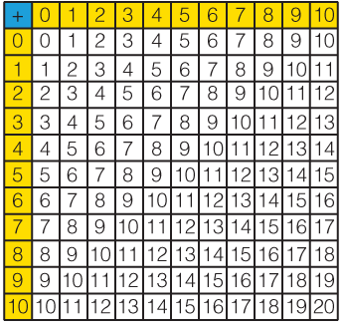Mathematics
Easy

Question

# Use fact patterns to complete each equation.1 + 3 = 42 + _____  = 4## 1234Hint:

## The correct answer is: 2

### Then we have given, 1 + 3 = 4 Now for , 2 + _ = 4So according to the pattern the first number of 4th row is answer, which is 2.Therefore , 2 + 2 = 4.The correct answer is 2 (option (b)).

In this question, just look the table to find the given addition . One equation is already given just follow the same pattern for the another equation .#### With Turito Foundation.#### Get an Expert Advice From Turito.